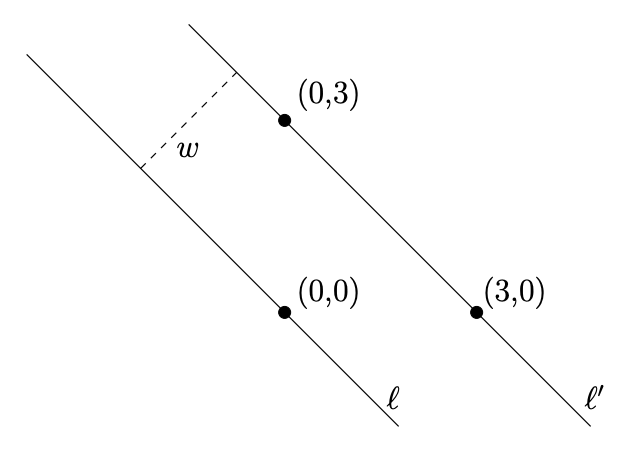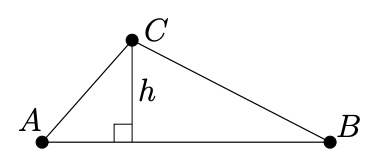시간 제한 메모리 제한 제출 정답 맞은 사람 정답 비율
2 초 512 MB 0 0 0 0.000%

## 문제

We consider a set $S$ of $n$ points in the plane. The width $w$ of $S$ is the minimum distance between two parallel lines that enclose $S$. For instance, in Figure 1, the setFigure 1: The width $w$ of a set of three points.

$S$ consists of $n = 3$ points $(0, 0)$, $(0, 3)$ and $(3, 0)$. The width is achieved by the two lines $\ell$ and $\ell'$, their distance is $w = 3\sqrt{2}/2 \simeq 2.12$. In this task, you are given a set of points in the input and you need to compute the integer part of $w^2$ and write it in the output. For instance, in Figure 1, we have $w = 3\sqrt{2}/2$, so $w^2 = 4.5$, and thus you need to output the integer part of $4.5$, which is $4$.

We give you a useful formula to help you solve this problem. Let $A = (x_a, y_a)$, $B = (x_b , y_b)$ and $C = (x_c, y_c)$ be three points. The height $h$ (see Figure 2) of the triangle $ABC$ is given by the following formula

$h = \sigma \frac{(x_a - x_c)(y_b-y_c) - (x_b-x_c)(y_a-y_c)}{\sqrt{(x_a-x_b)^2+(y_a-y_b)^2}}$

where $\sigma = 1$ if $ABC$ is counterclockwise (as in Figure 2) and $\sigma = −1$ if $ABC$ is clockwise.Figure 2: Triangle $ABC$.

Clearly, if all the points of $S$ lie on a straight line, the width $w$ is zero.

## 입력

The first input line contains the integer $n$, the number of points in $S$. Each of the next $n$ lines contains the $x$ coordinate and the $y$ coordinate of an input point separated by a space.

Note that the coordinates of the points are integers ranging from 0 to 199 inclusively. There are at most 100000 input points. A point may appear several times in the input file.

## 출력

The output file should contain the integer part of $w^2$.

## 예제 입력 1

3
0 0
3 0
0 3


## 예제 출력 1

4


## 예제 입력 2

4
0 0
3 0
0 3
3 0


## 예제 출력 2

4